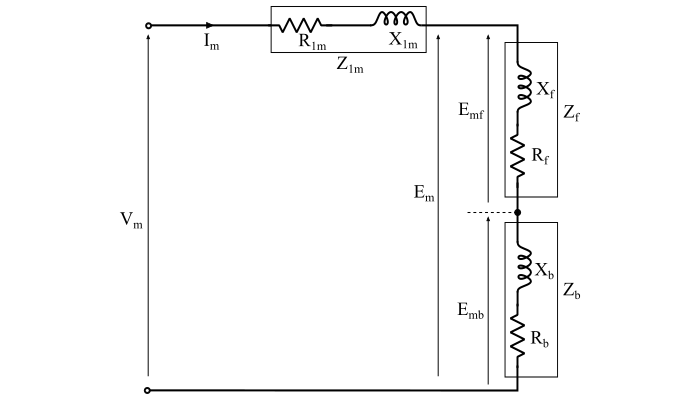# Performance Analysis of Single-Phase Single-Winding Induction Motor

The performance analysis of a single-phase, single-winding induction motor can be done with the help of the equivalent circuit that is shown in the figure. There are both forward and backward components of power and torque is present in the motor.Here, the torque of the backward field is in the opposite direction to that of the forward field and hence the total air-gap power in a single-phase induction motor is given by,

$$\mathrm{𝑃_{𝑔} = 𝑃_{𝑔𝑓} − 𝑃_{𝑔𝑏} … (1)}$$

Where, Pgf is the air-gap power for the forward field and is given by,

$$\mathrm{𝑃_{𝑔𝑓} = {{𝐼}^{2}_{𝑚}}\:𝑅_{𝑓}}$$

And Pgb is the air-gap power for the backward field and is given by,

$$\mathrm{𝑃_{𝑔𝑏} = {{𝐼}^{2}_{𝑚}}\:𝑅_{b}}$$

Therefore, the total air-gap power may also be given as,

$$\mathrm{𝑃_{𝑔} = {{𝐼}^{2}_{𝑚}}𝑅_{𝑓} − {{𝐼}^{2}_{𝑚}}𝑅_{b} = {{𝐼}^{2}_{𝑚}}(𝑅_{𝑓} − 𝑅_{𝑏} ) … (2)}$$

Now, the torque produced by the forward field is given by,

$$\mathrm{τ_{𝑓} =\frac{𝑃_{𝑔𝑓}}{𝜔_{𝑠}}=\frac{𝑃_{𝑔𝑓}}{2π 𝑛_{𝑠}}… (3)}$$

And the torque produced by the backward field is given by,

$$\mathrm{τ_{b} =\frac{𝑃_{𝑔𝑏}}{𝜔_{𝑠}}=\frac{𝑃_{𝑔b}}{2π 𝑛_{𝑠}}… (4)}$$

Where,

• ω𝑠 = Synchronous speed in radians per second, and

• 𝑛𝑠 = Synchronous speed in rps.

The resultant electromagnetic torque (τe) produced in the motor is equal to the difference between the forward torque (τf) and the backward torque (τb), i.e.,

$$\mathrm{τ_{𝑒} = τ_{𝑓} − τ_{𝑏}}$$

The rotor copper loss due to the forward field of a 1-phase induction motor is given by,

$$\mathrm{𝑃_{𝑟𝑐𝑢.𝑓} = 𝑠𝑃_{𝑔𝑓} … (5)}$$

And the rotor copper loss due to the backward field of a 1-phase induction motor is given by,

$$\mathrm{𝑃_{𝑟𝑐𝑢.𝑏} = (2 − 𝑠)𝑃_{𝑔𝑏} … (6)}$$

Therefore, the total rotor copper loss in a single-phase induction motor is given by,

$$\mathrm{𝑃_{𝑟𝑐𝑢} = 𝑠𝑃_{𝑔𝑓} + (2 − 𝑠)𝑃_{𝑔𝑏} … (7)}$$

The electrical power transformed into the mechanical power in a single-phase induction motor is given by,

$$\mathrm{𝑃_{𝑚} = ω τ_{𝑒} … (8)}$$

Where, ω is the angular speed of the rotor measured in radians per second.

Since,

$$\mathrm{ω = (1 − 𝑠) × ω_{𝑠}}$$

$$\mathrm{∴\:𝑃_{𝑚} = (1 − 𝑠) × ω_{𝑠}τ_{𝑒}}$$

$$\mathrm{\Rightarrow\:𝑃_{𝑚} = (1 − 𝑠) × 𝑃_{𝑔} = {𝐼^2_{𝑚}} (1 − 𝑠)(𝑅_{𝑓} − 𝑅_{𝑏 }) … (9)}$$

Therefore, the power available at the motor shaft is given by

$$\mathrm{𝑃_{out} = 𝑃_{𝑚} − 𝑃_{core\:loss} − 𝑃_{𝑓𝑤} − 𝑃_{stray\:losses}}$$

Where, Pfw is the friction and windage losses.

$$\mathrm{∵\:𝑃_{rot.} = 𝑃_{core\:loss} + 𝑃_{𝑓𝑤}}$$

$$\mathrm{∴\:𝑃_{out} = 𝑃_{𝑚} − 𝑃_{rot.} − 𝑃_{stray\:losses} … (10)}$$